### CONTRAST Statement

CONTRAST ’label’ row-description <,, row-description </ options>> ;

where a row-description is defined as follows:

effect values <, …, effect values>

The CONTRAST statement provides a mechanism for obtaining customized hypothesis tests. It is similar to the CONTRAST statement in PROC LOGISTIC and PROC GLM, depending on the coding schemes used with any classification variables involved.

The CONTRAST statement enables you to specify a matrix,, for testing the hypothesis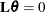, whereis the parameter vector. You must be familiar with the details of the model parameterization that PROC SURVEYLOGISTIC uses (for more information, see the PARAM= option in the section CLASS Statement). Optionally, the CONTRAST statement enables you to estimate each row,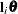, of
and test the hypothesis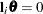. Computed statistics are based on the asymptotic chi-square distribution of the Wald statistic.

There is no limit to the number of CONTRAST statements that you can specify, but they must appear after the MODEL statement.

The following parameters can be specified in the CONTRAST statement:

label

identifies the contrast on the output. A label is required for every contrast specified, and it must be enclosed in quotes.

effect

identifies an effect that appears in the MODEL statement. The name INTERCEPT can be used as an effect when one or more intercepts are included in the model. You do not need to include all effects that are included in the MODEL statement.

values

are constants that are elements of thematrix associated with the effect. To correctly specify your contrast, it is crucial to know the ordering of parameters within each effect and the variable levels associated with any parameter. The Class Level Information table shows the ordering of levels within variables. The E option, described later in this section, enables you to verify the proper correspondence of values to parameters.

The rows ofare specified in order and are separated by commas. Multiple degree-of-freedom hypotheses can be tested by specifying multiple row-descriptions. For any of the full-rank parameterizations, if an effect is not specified in the CONTRAST statement, all of its coefficients in thematrix are set to 0. If too many values are specified for an effect, the extra ones are ignored. If too few values are specified, the remaining ones are set to 0.

When you use effect coding (by default or by specifying PARAM=EFFECT in the CLASS statement), all parameters are directly estimable (involve no other parameters).

For example, suppose an effect that is coded CLASS variable A has four levels. Then there are three parameters (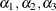) that represent the first three levels, and the fourth parameter is represented by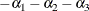To test the first versus the fourth level of A, you would test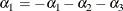or, equivalently,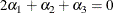which, in the form, is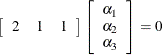Therefore, you would use the following CONTRAST statement:

contrast '1 vs. 4' A 2 1 1;


To contrast the third level with the average of the first two levels, you would test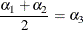or, equivalently,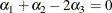Therefore, you would use the following CONTRAST statement:

contrast '1&2 vs. 3' A 1 1 -2;


Other CONTRAST statements are constructed similarly. For example:

contrast '1 vs. 2    '  A  1 -1  0;
contrast '1&2 vs. 4  '  A  3  3  2;
contrast '1&2 vs. 3&4'  A  2  2  0;
contrast 'Main Effect'  A  1  0  0,
A  0  1  0,
A  0  0  1;


When you use the less-than-full-rank parameterization (by specifying PARAM=GLM in the CLASS statement), each row is checked for estimability. If PROC SURVEYLOGISTIC finds a contrast to be nonestimable, it displays missing values in corresponding rows in the results. PROC SURVEYLOGISTIC handles missing level combinations of classification variables in the same manner as PROC LOGISTIC. Parameters corresponding to missing level combinations are not included in the model. This convention can affect the way in which you specify thematrix in your CONTRAST statement. If the elements ofare not specified for an effect that contains a specified effect, then the elements of the specified effect are distributed over the levels of the higher-order effect just as the LOGISTIC procedure does for its CONTRAST and ESTIMATE statements. For example, suppose that the model contains effects A and B and their interaction A*B. If you specify a CONTRAST statement involving A alone, thematrix contains nonzero terms for both A and A*B, since A*B contains A.

The degrees of freedom is the number of linearly independent constraints implied by the CONTRAST statement—that is, the rank of.

You can specify the following options after a slash (/):

ALPHA=value

sets the confidence level for confidence limits. The value of the ALPHA= option must be between 0 and 1, and the default value is 0.05. A confidence level ofproduces% confidence limits. The default of ALPHA=0.05 produces 95% confidence limits.

E

requests that thematrix be displayed.

ESTIMATE=keyword

requests that each individual contrast (that is, each row,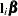, of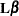) or exponentiated contrast (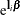) be estimated and tested. PROC SURVEYLOGISTIC displays the point estimate, its standard error, a Wald confidence interval, and a Wald chi-square test for each contrast. The significance level of the confidence interval is controlled by the ALPHA= option. You can estimate the contrast or the exponentiated contrast (), or both, by specifying one of the following keywords:

PARM

specifies that the contrast itself be estimated

EXP

specifies that the exponentiated contrast be estimated

BOTH

specifies that both the contrast and the exponentiated contrast be estimated

SINGULAR=value

tunes the estimability checking. Ifis a vector, define ABS() to be the largest absolute value of the elements of. For a row vectorof the matrix, define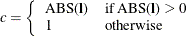If ABS(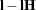) is greater than c*value, then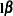is declared nonestimable. The
matrix is the Hermite form matrix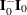, where
represents a generalized inverse of the information matrix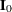of the null model. The value must be between 0 and 1; the default is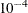.